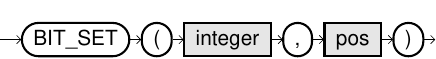# BIT_SET

## Purpose

This function sets a certain bit of a numerical value. The position parameter starts from 0, which is the lowest bit.

## Syntax

bit_set::=## Usage Notes

• Bit functions are limited to 64 bits, which is positive numbers between 0 and 18446744073709551615.
• The value pos may be between 0 and 63.
• The result data type is DECIMAL(20,0).

## Example

``SELECT BIT_SET(8,0);``